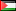##### Analytical and Numerical Investigation of the Fredholm Integral Equation for the Heat Radiation Problem
Publication Type
Original research
Authors
• Naji Qatanani
• Monika Schulz
Fulltext

This article deals with the mathematical and the numerical aspects of the Fredholm integral equation of the second kind arising as a result of the heat energy exchange inside a convex and non-convex enclosure geometries. Some mathematical results concerning the integral operator are presented. The Banach fixed point theorem is used to guarantee the existence and the uniqueness of the solution of the integral equation. For the non-convex geometries the visibility function has to be taken into consideration, then a geometrical algorithm is developed to provide an efficient detection of the shadow zones. For the numerical simulation of the integral equation we use the boundary element method based on the Galerkin discretization scheme. Some iterative methods for the discrete radiosity equation are implemented. Several two- and three-dimensional numerical test cases for convex and non-convex geometries are included. This give concrete hints which iterative scheme might be more useful for such practical applications.

Journal
Title
Applied Mathematics and Computation Volume 175, Issue 1, 1 April 2006, Pages 149–170
Publisher
--
Publisher Country
PalestinePublication Type
Both (Printed and Online)
Volume
--
Year
2006
Pages
--